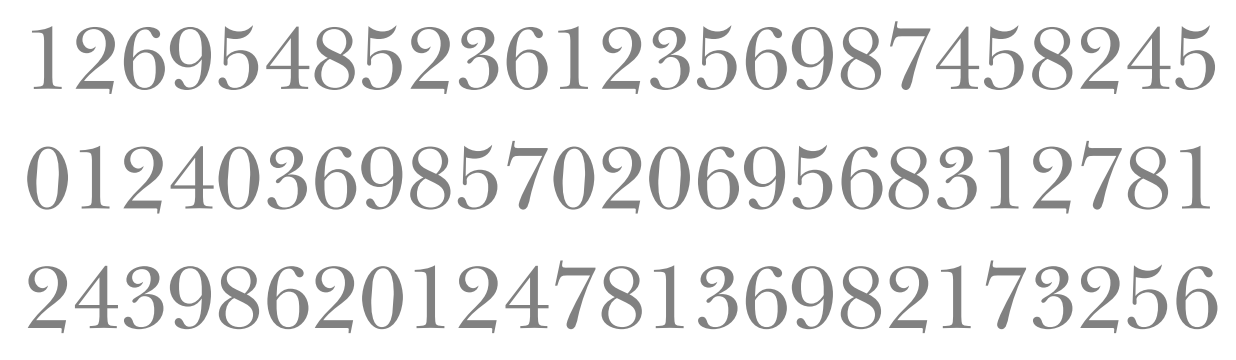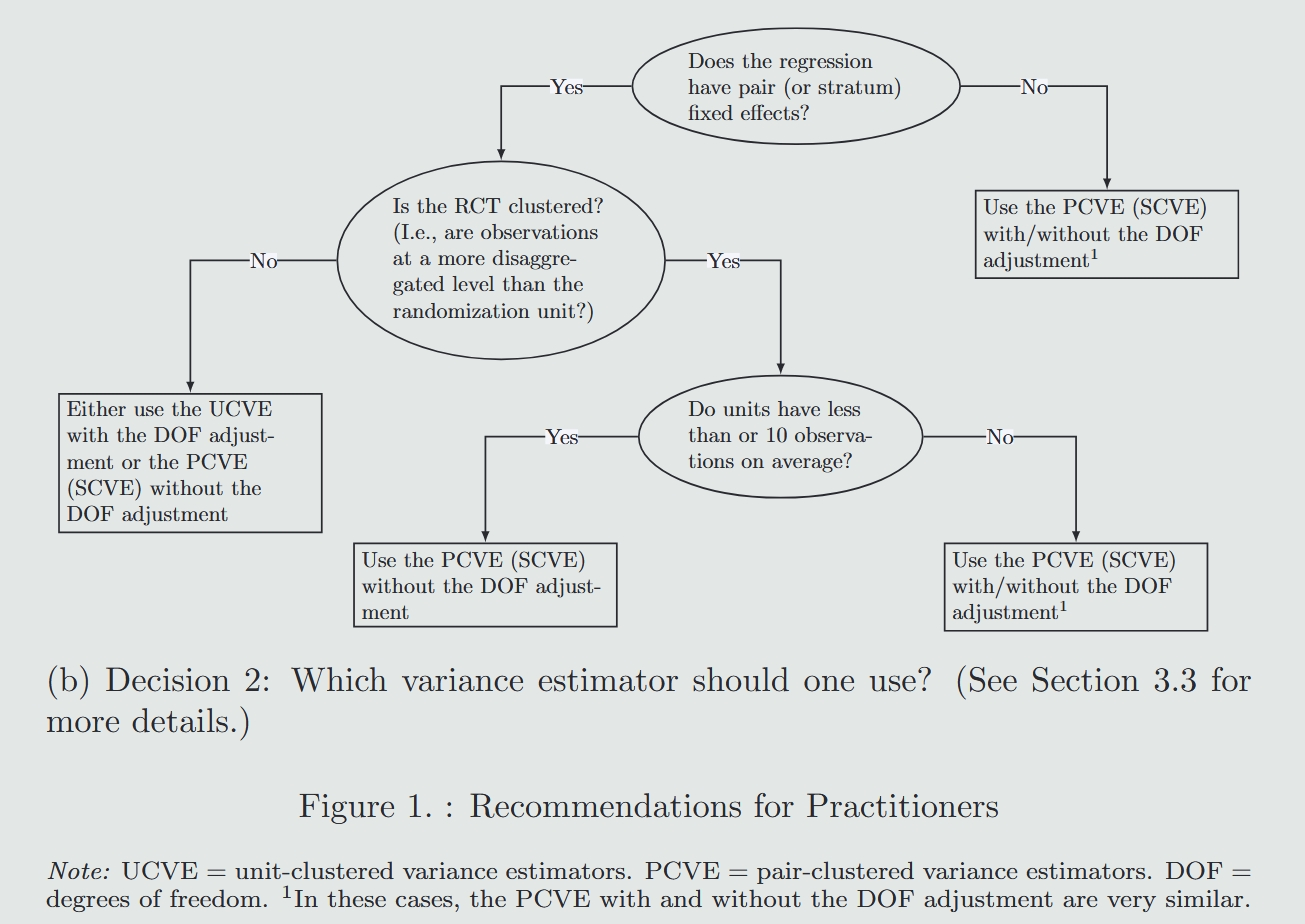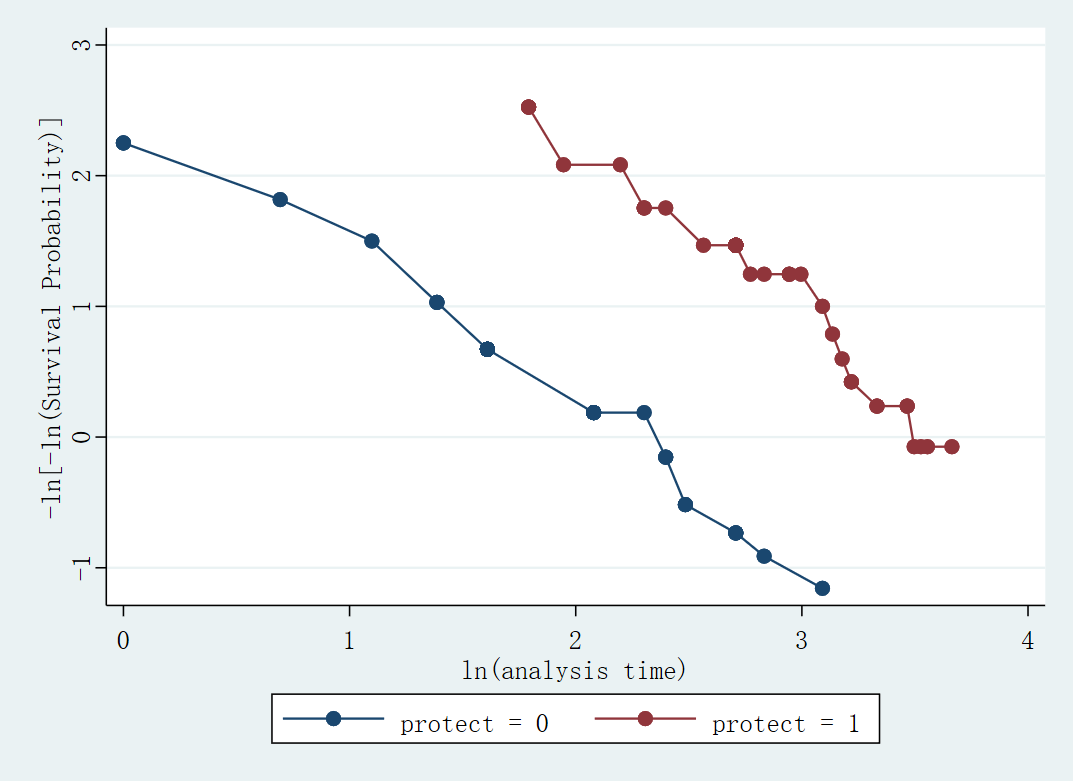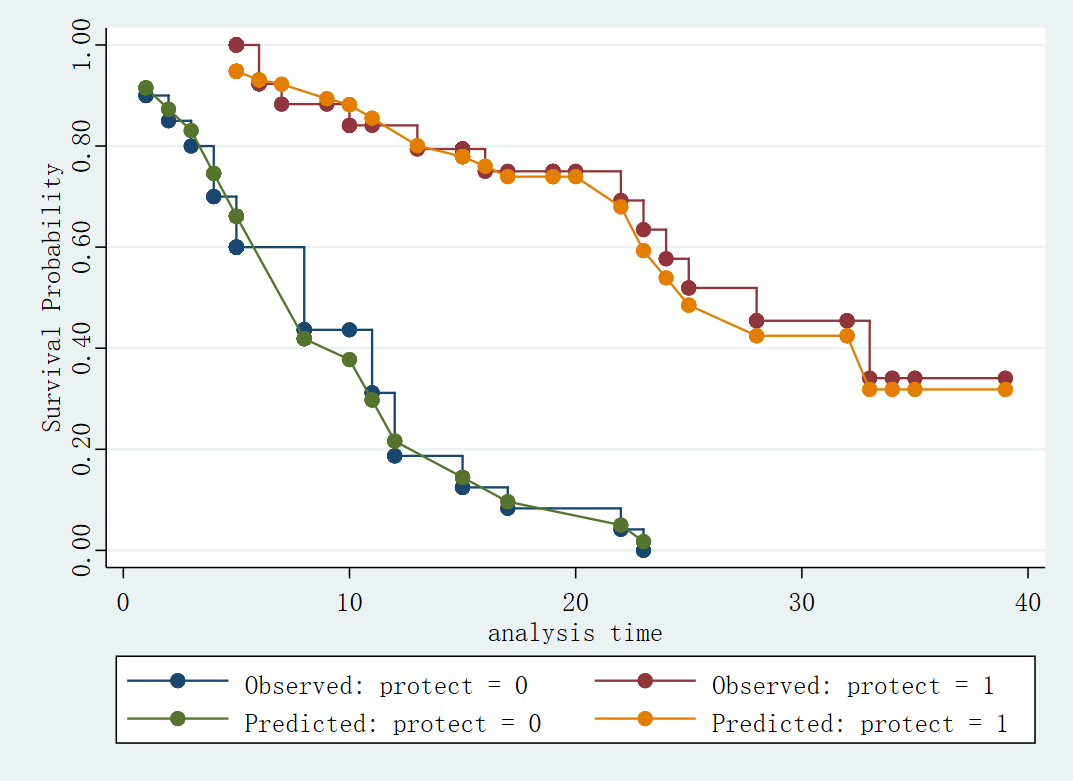# Stata: 生存分析一文读懂

Stata 连享会   主页 || 视频 || 推文

Email: lqqflora@163.com

## 1. 生存分析简介

• 失业持续的时间
• 初创企业存活时间
• 从开始筹备到顺利 IPO 所需的时间

• 农村劳动力向城市流动的影响因素分析（张世伟, 赵亮, 2009）
• 企业生存分析 (吴斌, 2006; 逯宇铎等，2013; 许家云，毛其淋，2016; 刘海洋等, 2017)
• 扭曲工资与企业成长 (吴先明, 2017)
• 企业新产品创新 (毛其淋,许加云, 2014; 毛其淋, 许加云, 2015)
• 企业出口 (毛其淋, 盛斌, 2013)
• 中国进出口贸易持续期 (邵军, 2011; 谭晶荣, 童晓乐, 2014; 何树全, 张秀霞, 2011; 赵瑞丽等, 2016)
• 上市公司 "ST特别处理" (吕长, 江赵岩, 2004)
• 企业财务困境预警 (宋雪枫,杨朝军,2006; 陆志明等，2007; 王晓鹏等, 2007; 陆志明等, 2007; 过新伟, 胡晓, 2012; 李宏伟,2019)
• 网络贷款违约风险 (李思瑶等, 2016)
• 女性生育间隔 (靳永爱等, 2019)
• ……

### 1.1 基本介绍

• 定性变量状态变化所花费的时间, 比如离婚、晋升、死亡
• 定量变量出现急剧变化所经历的时间, 比如人口总和出生率锐减 (见 Figure 1)
• 定量变量超过某个阈值所需要的时间, 比如将体重减到健康标准 (见 Figure 2)Figure 1: Quantitative variables change sharplyFigure2: Quantitative variables cross a threshold

### 1.2 生存分析解决的问题

#### 例子

• 研究方法 A： 用二元被解释变量 (逮捕 = 1, 没有逮捕 = 0) 对解释变量进行线性回归；

• 局限： 应该使用逻辑回归 (Logistic Model); 没有充分利用信息; 不能有效处理工作状态这一变量(此变量随时间而变动)
• 研究方法 B： 把被解释变量定义为犯人从释放到第一次被捕的时间，再进行线性回归。

• 局限： 出现有些个体没有被逮捕或者在追踪期以后被逮捕却被记录成 2 年的情况 (censoring), 并且依旧无法概括随时间变动的解释变量。

#### 截堵 (censoring)

• 右截堵: 将 $c$ 作为被解释变量的上限，但是存在一些观测值 $T$ 大于 $c$。例如: 对一组年龄为 30 岁的女性进行采访, 关注的事件是初婚时间。采访中提出的问题是「结婚时的年龄 ($T$)」。本例中 $c=30$。然而，若样本中包含部分未婚女性, 她们的结婚年龄一定会超过 30 岁, 但由于抽样方法的局限，其具体结婚年龄无法在访谈中得知。

• 左截堵: 将 $c$ 作为被解释变量的下限，但是存在一些观测值 $T$ 小于 $c$。例如: 对一组年龄在 20 岁及以上的女性进行采访, 关注的事件同样是初婚年龄。最开始提的问题为是否结婚, 这里的 $c$ 为 20。 但是有一些 20 岁的女性已经结婚，则她们的结婚年龄一定小于 20, 具体是多少无法从提问中知晓。

• 区间截堵: 将 ${c}_{1}$ 和 ${c}_{2}$ 分别作为被解释变量的下限和上限, 存在观测值 $T$ 小于 ${c}_{1}$, 也存在观测值 $T$ 大于 ${c}_{2}$。 例如: 对一组年龄在 20 岁到 30 岁之间中国女性进行采访，问她们第一次生育的年龄, 这里 ${c}_{1}$ 等于 20 (中国女性合法结婚年龄, 婚后生孩子才是合法的)， ${c}_{2}$ 为 30。 30 岁的女性还没有生育，则其生育年龄一定超过 30, 对于 20 岁以前生孩子的女性，考虑到隐私及法律问题，并没有回答具体生育年龄, 只是说了已经有孩子, 则她们的生育年龄一定小于 20。

## 2. 生存分析模型

### 2.2 风险函数的类型

#### 冈珀茨模型 (Gompertz model)

• 当 $\alpha <0$ 时，单调递减;
• 当 $\alpha =0$ 时，变为指数模型的风险函数;
• 当 $\alpha >0$ 时，单调递增。

#### 威布尔模型 (Weibull model)

• 当 $\alpha <0$ 时，单调递减;
• 当 $\alpha =0$ 时，变为指数模型的风险函数;
• 当 $\alpha >0$ 时，单调递增。

#### 小结

• 指数模型：$\mathrm{log}\lambda \left(t\right)={\beta }_{0}+{\beta }_{1}{x}_{1}+{\beta }_{2}{x}_{2}$
• 冈珀茨模型：$\mathrm{log}\lambda \left(t\right)={\beta }_{0}+{\beta }_{1}{x}_{1}+{\beta }_{2}{x}_{2}+\alpha t$
• 威布尔模型：$\mathrm{log}\lambda \left(t\right)={\beta }_{0}+{\beta }_{1}{x}_{1}+{\beta }_{2}{x}_{2}+\alpha \mathrm{log}t$

:

• $\beta$ 为半弹性, 即某个解释变量增加 1 个单位，将导致风险函数平均增加百分之 $\beta$
• ${e}^{\beta }$ 为风险比率 (Hazard Ratio), 即某个解释变量变量增加 1 单位, 将导致新风险比率变为原来的 ${e}^{\beta }$ 倍。
• 另外, 不含解释变量 $X$ 的风险函数通常被称为 基准风险${\lambda }_{0}\left(t\right)$

### 2.3 加速失效时间模型

#### 哪些具体的模型可以视为加速失效时间模型 ?

Cameron and Trival (2005) 总结有 5 种加速失效时间模型, 其中指数模型和威布尔模型既是比例风险模型 (PH) 又是加速失效时间模型 (AFT).

![Table1： 参数模型](x https://fig-lianxh.oss-cn-shenzhen.aliyuncs.com/Survival-Analysis-AFT.png)

Table 17.5. Standard Parametric Models and Their Hazard and Survivor Functions

Parametric Model Hazard Function Survivor Function Type
Exponential $\gamma$ $\mathrm{exp}\left(-\gamma t\right)$ PH, AFT
Weibull $\gamma \alpha {t}^{\alpha -1}$ $\mathrm{exp}\left(-\gamma {t}^{\alpha }\right)$ PH, AFT
Generalized Weibull $\gamma \alpha {t}^{\alpha -1}S\left(t{\right)}^{-\mu }$ ${\left[1-\mu \gamma {t}^{\alpha }\right]}^{1/\mu }$ PH
Gompertz $\gamma \mathrm{exp}\left(\alpha t\right)$ $\mathrm{exp}\left(-\left(\gamma /\alpha \right)\left({e}^{\alpha t}-1\right)\right)$ PH
Log-normal $\frac{\mathrm{exp}\left(-\left(\mathrm{ln}t-\mu {\right)}^{2}/2{\sigma }^{2}\right)}{t\sigma \sqrt{2\pi }\left[1-\mathrm{\Phi }\left(\mathrm{ln}t-\mu \right)/\sigma \right)\right]}$ $1-\mathrm{\Phi }\left(\left(\mathrm{ln}t-\mu \right)/\sigma \right)$ AFT
Log-logistic $\alpha {\gamma }^{\alpha }{t}^{\alpha -1}/\left[\left(1+\left(\gamma t{\right)}^{\alpha }\right)\right]$ $1/\left[1+\left(\gamma t{\right)}^{\alpha }\right]$ AFT
Gamma $\frac{\gamma \left(\gamma t{\right)}^{\alpha -1}\mathrm{exp}\left[-\left(\gamma t\right)\right]}{\mathrm{\Gamma }\left(\alpha \right)\left(1-I\left(\alpha ,\gamma t\right)\right]}$ $1-I\left(\alpha ,\gamma t\right)$ AFT

Source: Cameron and Trival (2005), Table 17.5

## 3. 生存分析的基本步骤及 Stata 命令

• 第一步 设定生存分析数据
• 第二步 画生存函数、累积风险函数和风险函数
• 第三步 进行参数回归及非参数回归
• 第四步 比例风险假定的检验

• 关注事件 为骨折 (fracture)
• 持续时间 为开始被调查到发生骨折(fracture = 1) 的时间, 同时也包括发生过骨折以后恢复了再次发生骨折的时间 (被试会被调查几个阶段，不同阶段之间会有时间间隔)
• 解释变量 包括：年龄 (age)、血钙（calcium) 和 穿戴防护装置 (protect)。

### 3.1 将数据设定为生存分析格式

``````. use http://www.stata-press.com/data/cggm3/hip, clear //调用 hip.dta 数据
. describe //对数据集进行描述
*-----------使用 describe 命令 table2 ------
Contains data from http://www.stata-press.com/data/cggm3/hip.dta
obs:           106                          hip fracture study
vars:             7                          30 Jan 2009 11:58
size:         1,060
-------------------------------------------------------------------
storage   display    value
variable name   type    format     label      variable label
-------------------------------------------------------------------
id              byte    %4.0g                 patient ID
time0           byte    %5.0g                 begin of span
time1           byte    %5.0g                 end of span
fracture        byte    %8.0g                 fracture event
protect         byte    %8.0g                 wears device
age             byte    %4.0g                 age at enrollment
calcium         float   %8.0g                 blood calcium level
-------------------------------------------------------------------
``````

#### stset 命令

stset 命令将数据设定为适合生存分析的形式, 其语法为，

``````. stset tvar, failure(fail) id(idvar) origin(time torig)
``````

• `tvar` 为结束时间
• `failure(fail)` 设定关注事件, 默认 `fail==1` 为关注事件发生
• `id(idvar)` 设定样本中的用以标示每个观察值的变量
• `origin(time torig)` 设定 torig 为开始时间, 默认为 0。

``````. stset time1, origin(time time0) id(id) failure(fracture == 1)
*------------使用 stset 命令 table3 -------

id:  id
failure event:  fracture == 1
obs. time interval:  (time1[_n-1], time1]
exit on or before:  failure
t for analysis:  (time-origin)
origin:  time time0

--------------------------------------------------------------------------
106  total observations
0  exclusions
--------------------------------------------------------------------------
106  observations remaining, representing
48  subjects
31  failures in single-failure-per-subject data
744  total analysis time at risk and under observation
at risk from t =         0
earliest observed entry t =         0
last observed exit t =        39
``````

• _st = 1 表示该观测值的数据会被使用,
• _d 表示在一个记录中是否发生过关注事件,
• _t 代表一个记录的结束时间,
• _t0 代表一个记录的开始时间。

#### stdescribe 命令

`stdescribe` 命令是在 stset 命令之后使用的, 用于描述生存分析数据的基本特征

``````. stdescribe
*------------使用 stdescribe 命令 table4 -------
failure _d:  fracture == 1
analysis time _t:  (time1-origin)
origin:  time time0
id:  id

|-------------- per subject --------------|
Category                   total        mean         min     median        max
------------------------------------------------------------------------------
no. of subjects               48
no. of records               106    2.208333           1          2          3

(first) entry time                         0           0          0          0
(final) exit time                       15.5           1       12.5         39

subjects with gap              0
time on gap if gap             0           .           .          .          .
time at risk                 744        15.5           1       12.5         39

failures                      31    .6458333           0          1          1
------------------------------------------------------------------------------
``````

### 3.2 描述性分析 (非参数分析)

#### 生存函数图

Kaplan-Meier 估计量大致等于样本存活 (关注事件未发生) 时间超过 $t$ 的观测值数目占样本总体观测值的数目比值, 此方法无需对数据的分布做假设、也不需要进行参数估计, 故被称为非参数分析。

``````*-----使用 sts graph 命令 figure5 -----
//数据已设置成生存分析数据
. use http://www.stata-press.com/data/cggm3/hip2, clear
. sts graph  //生存分析图
. graph export figure5.png
``````

``````*-----使用 sts graph 命令 figure6-----
* 以是否穿防护装置分组画生存分析图
* plot2opts(lp("-"))表示第二个图用虚线表示
. sts graph, by(protect) plot2opts(lp("-"))
. graph export figure6.png
``````

#### 累积风险函数图

Nelson-Aalen 累积风险估计量是对局部风险率加总得出的，估计量为

``````*-----使用 sts graph 命令 figure7-----
sts graph, cumhaz ci //cumhaz 选项表示画累积风险函数, ci 显示 95% 的置信区间。
graph export figure7.png
``````

#### 风险函数图

``````*-----使用 sts graph 命令 figure8-----
sts graph, cumhaz ci //cumhaz by(protect)选项表示画累积风险函数, ci 显示 95% 的置信区间。
graph export figure7.png
``````

### 3.3 模型估计

#### 比例风险模型

``````. streg protect age calcium, nohr nolog dist(weib)
*- dist(weib) 代表威布尔回归的风险函数,
*- dist(e) 为指数回归，dist(gom) 为刚珀茨回归，
*- nohr 显示回归系数而不显示风险比率

*-----使用 streg 命令 table5-----
failure _d:  fracture
analysis time _t:  time1
id:  id

Weibull PH regression

No. of subjects =           48                  Number of obs    =         106
No. of failures =           31
Time at risk    =          714
LR chi2(3)       =       35.22
Log likelihood  =   -41.687862                  Prob > chi2      =      0.0000

------------------------------------------------------------------------------
_t |      Coef.   Std. Err.      z    P>|z|     [95% Conf. Interval]
-------------+----------------------------------------------------------------
protect |  -2.192387   .4078599    -5.38   0.000    -2.991778   -1.392996
age |   .0801079   .0550936     1.45   0.146    -.0278735    .1880893
calcium |  -.1744454   .2194006    -0.80   0.427    -.6044626    .2555718
_cons |  -7.787834   5.734033    -1.36   0.174    -19.02633    3.450665
-------------+----------------------------------------------------------------
/ln_p |   .5207567   .1369941     3.80   0.000     .2522531    .7892602
-------------+----------------------------------------------------------------
p |   1.683301   .2306023                      1.286922    2.201767
1/p |   .5940709   .0813842                      .4541807     .777048
------------------------------------------------------------------------------
``````

``````*-----使用 stcurve 命令 figure 9-----
. stcurve, hazard //画风险函数图
``````

#### 加速时间失效模型 (AFT)

``````*-----使用 streg 命令 table6-----
.  streg protect age calcium,  nolog dist(logn)

failure _d:  fracture
analysis time _t:  time1
id:  id

Lognormal AFT regression

No. of subjects =           48                  Number of obs    =         106
No. of failures =           31
Time at risk    =          714
LR chi2(3)       =       35.50
Log likelihood  =   -41.758706                  Prob > chi2      =      0.0000

------------------------------------------------------------------------------
_t |      Coef.   Std. Err.      z    P>|z|     [95% Conf. Interval]
-------------+----------------------------------------------------------------
protect |    1.44531   .2495669     5.79   0.000     .9561681    1.934452
age |  -.0639809    .041565    -1.54   0.124    -.1454468    .0174849
calcium |   .0692953   .1668585     0.42   0.678    -.2577412    .3963319
_cons |    5.74614   4.416337     1.30   0.193    -2.909722      14.402
-------------+----------------------------------------------------------------
/lnsigma |   -.294334   .1271106    -2.32   0.021    -.5434661   -.0452018
-------------+----------------------------------------------------------------
sigma |   .7450276   .0947009                      .5807319    .9558046
------------------------------------------------------------------------------
``````

``````*-----使用 stcurve 命令 figure 10-----
. stcurve, hazard //画风险函数图
``````

#### Cox PH 模型

``````. stcox protect age calcium,r nohr nolog
*-----使用 streg 命令 table 7-----
Cox regression -- Breslow method for ties

No. of subjects      =           48             Number of obs    =         106
No. of failures      =           31
Time at risk         =          714
Wald chi2(3)     =       31.21
Log pseudolikelihood =   -82.062396             Prob > chi2      =      0.0000

(Std. Err. adjusted for 48 clusters in id)
------------------------------------------------------------------------------
|               Robust
_t |      Coef.   Std. Err.      z    P>|z|     [95% Conf. Interval]
-------------+----------------------------------------------------------------
protect |  -2.242575   .4328035    -5.18   0.000    -3.090855   -1.394296
age |   .0676642   .0438904     1.54   0.123    -.0183594    .1536878
calcium |  -.2114179   .1600249    -1.32   0.186    -.5250609    .1022251
------------------------------------------------------------------------------
``````

``````*-----使用 stcurve 命令 figure 11-----
. stcurve, hazard //画风险函数图
``````

### 3.4 模型的检验

#### 对数 - 对数图

``````*-----使用 stphplot 命令 figure 12-----
. stphplot, by(protect)
``````Figure 12: 基于变量 protect 的对数 - 对数图

#### 观测 - 预测图

``````*-----使用 stcoxkm 命令 figure 13-----
. stcoxkm, by(protect)
``````Figure 13: 基于变量 protect 的观测 - 预测图

#### 舍恩尔德残差图

``````quitely stcox protect age calcium,r nohr nolog
estat phtest, detail

*-----使用 estat phtest命令 table8 -----
Test of proportional-hazards assumption

Time:  Time
----------------------------------------------------------------
|       rho            chi2       df       Prob>chi2
------------+---------------------------------------------------
protect     |      0.00064         0.00        1         0.9974
age         |     -0.10011         0.17        1         0.6828
calcium     |      0.00017         0.00        1         0.9997
------------+---------------------------------------------------
global test |                      0.26        3         0.9674
----------------------------------------------------------------
``````

``````*-----使用 estat phtest命令 figure14 -----
. estat phtest, plot(protect)
``````

### 3.5 国内研究实例

【Data & Stata Files】，右击另存即可。

Source: 吴先明等 (2017)，表 3. Data & Stata Files

``````*-吴先明等 (2017) 表 3 - Stata 命令 - 基于 Cox PH 的回归分析
*-完整文件地址：http://www.ciejournal.org/Magazine/show/?id=51567

/////以企业进入成长成熟期作为兴趣事件构建生存分析数据/////
stset year, id(panelid2) failure(life==1) origin(xkysj)

/////表3基于Cox PH模型的回归分析-从初创期到成长成熟期部分/////
stcox wdist  pdens_100  size  lever   cdens_100  subs roa export state  foreig  ///
HHI grow  mature  decline , nohr
est store model1

stcox wdist  pdens_100 wdist_pdens_100  size  lever   cdens_100  subs roa export ///
state  foreig    HHI grow  mature  decline , nohr
est store model2

stcox wdist  pdens_100 wdist_pdens_100  size  lever   cdens_100  subs roa export ///
state  foreig    HHI grow  mature  decline _Iindu* , nohr
est store model3

stcox wdist  pdens_100 wdist_pdens_100  size  lever   cdens_100  subs roa export ///
state  foreig    HHI grow  mature  decline _I* , nohr
est store model4

stset, clear

/////以企业进入衰退期作为兴趣事件
/////但以企业进入成长成熟期作为进入研究的时间，构建生存分析数据
stset year, id(panelid2) failure(life==2) origin(xkysj) enter(life==1)

/////表3基于Cox PH模型的回归分析-从成长成熟期到衰退期部分/////
stcox wdist  pdens_100  size  lever   cdens_100  subs roa export state  foreig ///
HHI grow  mature  decline , nohr
est store model5

stcox wdist  pdens_100 wdist_pdens_100  size  lever   cdens_100  subs roa export ///
state  foreig    HHI grow  mature  decline , nohr
est store model6

stcox wdist  pdens_100 wdist_pdens_100  size  lever   cdens_100  subs roa export ///
state  foreig    HHI grow  mature  decline _Iindu* , nohr
est store model7

stcox wdist  pdens_100 wdist_pdens_100  size  lever   cdens_100  subs roa export ///
state  foreig    HHI grow  mature  decline _I* , nohr
est store model8

///导出表3基于Cox PH模型的回归分析
outreg2 [*] using 表3基于Cox PH模型的回归分析.xls, replace
``````

## 参考文献

• Cameron, A.C. and P.K. Trivedi. 2005, Microeconometrics Methods and Applications. Cambriage University Press, New York, NY.
• Cleves, M., W. Gould, and R. Gutierrez. 2002. An Introduction to Survival Analysis Using Stata. College Station, TX: Stata Press. [PDF]
• Survival Analysis Using Stata - Statistical Horizons. [PDF]
• 陈强.高级计量经济学及 Stata 应用 [M] . 高等教育出版社, 2014.
• 陈勇兵,钱意,张相文.中国进口持续时间及其决定因素[J].统计研究,2013,30(02):49-57.
• 过新伟,胡晓.CEO变更与财务困境恢复——基于ST上市公司"摘帽"的实证研究[J].首都经济贸易大学学报,2012,14(03):47-54.
• 何树全,张秀霞.中国对美国农产品出口持续时间研究[J].统计研究,2011,28(02):34-38.
• 李宏伟.基于生存分析的上市公司财务困境时间效应研究[J].财会通讯,2019(32):31-35.
• 李思瑶,王积田,柳立超.基于生存分析的P2P网络借贷违约风险影响因素研究[J].经济体制改革,2016(06):156-160.
• 刘海洋,林令涛,黄顺武.地方官员变更与企业兴衰——来自地级市层面的证据[J].中国工业经济,2017(01):62-80.
• 逯宇铎,于娇,刘海洋.集聚经济是否影响了企业生命周期——基于企业退出行为视角[J].财经科学,2013(10):60-70.
• 陆志明,何建敏,姜丽莉.基于生存分析模型的企业财务困境预测[J].统计与决策,2007(21):174-176.
• 吕长江,赵岩.中国上市公司特别处理的生存分析[J].中国会计评论,2004(02):311-338.
• 毛其淋,盛斌.贸易自由化、企业异质性与出口动态——来自中国微观企业数据的证据[J].管理世界,2013(03):48-65+68+66-67.
• 毛其淋,许家云.中国对外直接投资促进抑或抑制了企业出口?[J].数量经济技术经济研究,2014,31(09):3-21.
• 毛其淋,许家云.中国企业对外直接投资是否促进了企业创新[J].世界经济,2014,37(08):98-125.
• 蒋灵多,陈勇兵.出口企业的产品异质性与出口持续时间[J].世界经济,2015,38(07):3-26.
• 靳永爱,陈杭,李芷琪.流动与女性生育间隔的关系——基于2017年全国生育状况抽样调查数据的实证分析[J].人口研究,2019,43(06):3-19.
• 宋雪枫,杨朝军.财务危机预警模型在商业银行信贷风险管理中的应用[J].国际金融研究,2006(05):14-20.
• 谭晶荣,童晓乐.中国与金砖国家贸易关系持续时间研究[J].国际贸易问题,2014(04):90-100.
• 王晓鹏,何建敏,马立成.Cox模型在企业财务困境预警中的应用[J].价值工程,2007(11):4-8.
• 吴冰.生存分析及其应用:以创业研究为例[J].上海交通大学学报(哲学社会科学版),2006(03):63-65+75.
• 吴先明,张楠,赵奇伟.工资扭曲、种群密度与企业成长:基于企业生命周期的动态分析[J].中国工业经济,2017(10):137-155.
• 许家云,毛其淋.政府补贴、治理环境与中国企业生存[J].世界经济,2016,39(02):75-99.
• 张世伟,赵亮.农村劳动力流动的影响因素分析——基于生存分析的视角[J].中国人口·资源与环境,2009,19(04):101-106.
• 赵瑞丽,孙楚仁,陈勇兵.最低工资与企业出口持续时间[J].世界经济,2016,39(07):97-120.

## Appendix 本文涉及的 Stata 代码

``````use http://www.stata-press.com/data/cggm3/hip, clear //调用 hip.dta 数据
describe //对数据集进行描述
stset time1, origin(time time0) id(id) failure(fracture == 1) //设定数据
stdescribe //在 stset 命令之后使用的, 用于描述生存分析数据的基本特征

*-----使用 sts graph 命令 figure5 -----
use http://www.stata-press.com/data/cggm3/hip2, clear //数据已设置成生存分析数据
sts graph  //生存分析图
graph export figure5.png

*-----使用 sts graph 命令 figure6-----
sts graph, by(protect) plot2opts(lp("-")) //以是否穿防护装置分组画生存分析图  plot2opts(lp("-"))表示第二个图用虚线表示
graph export figure6.png

*-----使用 sts graph 命令 figure7-----
sts graph, cumhaz ci //cumhaz 选项表示画累积风险函数, ci 显示 95% 的置信区间。
graph export figure7.png

*-----使用 sts graph 命令 figure8-----
sts graph, cumhaz ci //cumhaz by(protect)选项表示画累积风险函数, ci 显示 95% 的置信区间。
graph export figure7.png

*-----使用 streg 命令 table5-----
streg protect age calcium, nohr nolog dist(weib)
* dist(weib)代表威布尔回归的风险函数,
* dist(e)指数回归，
* dist(gom)刚珀茨回归，
* nohr显示回归系数而不显示风险比率

*-----使用 stcurve 命令 figure 9-----
stcurve, hazard //画风险函数图

*-----使用 stcurve 命令 figure 10-----
stcurve, hazard //画风险函数图

*-----使用 streg 命令 table6-----
streg protect age calcium,  nolog dist(logn)

*-----使用 streg 命令 table 7-----
. stcox protect age calcium,r nohr nolog

*-----使用 stcurve 命令 figure 11-----
stcurve, hazard //画风险函数图

*-----使用 stphplot 命令 figure 12-----
. stphplot, by(protect)

*-----使用 stcoxkm 命令 figure 13-----
. stcoxkm, by(protect)

*-----使用 estat phtest命令 table8 -----
quitely stcox protect age calcium,r nohr nolog
estat phtest, detail

*-----使用 estat phtest命令 figure14 -----
estat phtest, plot(protect)

``````

## 相关课程

http://lianxh.duanshu.com

### 课程一览

Stata数据清洗 游万海 直播, 2 小时，已上线

Note: 部分课程的资料，PPT 等可以前往 连享会-直播课 主页查看，下载。

#### 关于我们

• Stata连享会 由中山大学连玉君老师团队创办，定期分享实证分析经验。直播间 有很多视频课程，可以随时观看。
• 连享会-主页知乎专栏，300+ 推文，实证分析不再抓狂。
• 公众号推文分类： 计量专题 | 分类推文 | 资源工具。推文分成 内生性 | 空间计量 | 时序面板 | 结果输出 | 交乘调节 五类，主流方法介绍一目了然：DID, RDD, IV, GMM, FE, Probit 等。
• 公众号关键词搜索/回复 功能已经上线。大家可以在公众号左下角点击键盘图标，输入简要关键词，以便快速呈现历史推文，获取工具软件和数据下载。常见关键词：`课程, 直播, 视频, 客服, 模型设定, 研究设计, stata, plus, 绘图, 编程, 面板, 论文重现, 可视化, RDD, DID, PSM, 合成控制法`